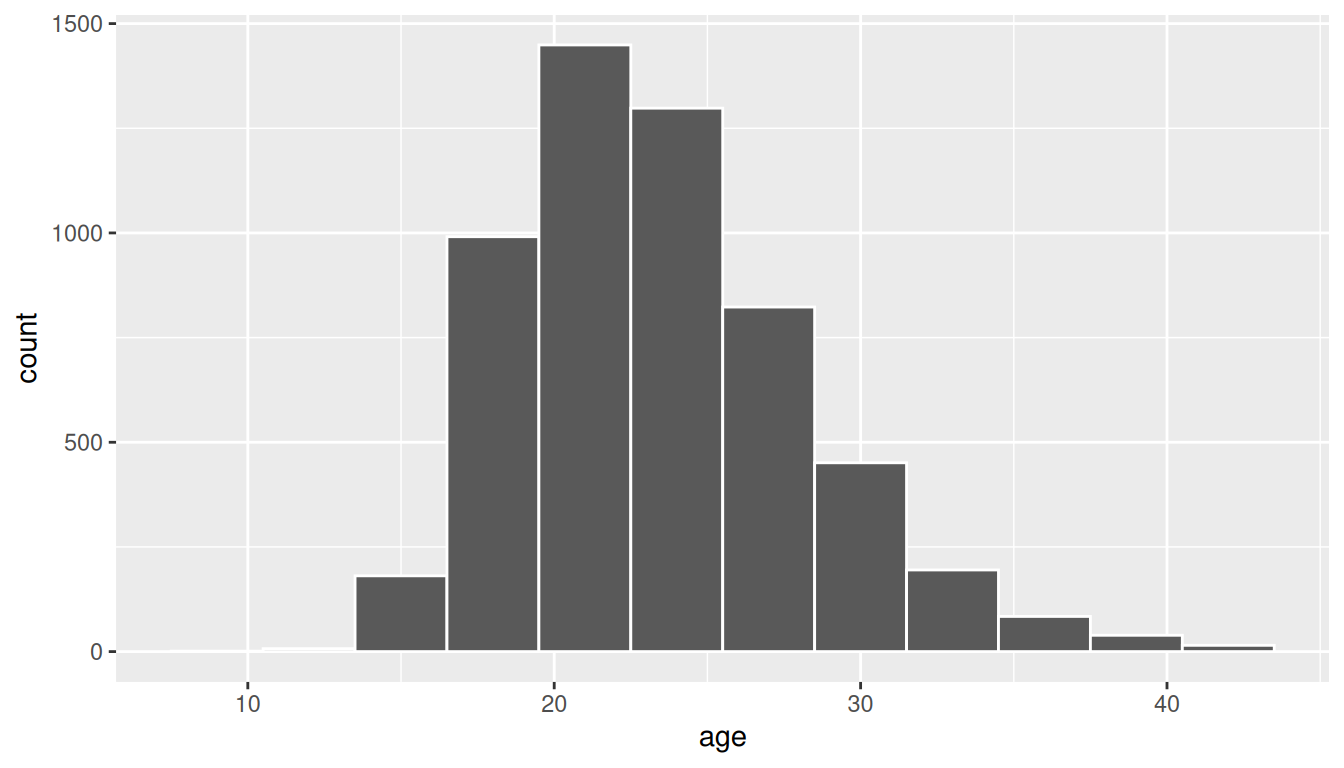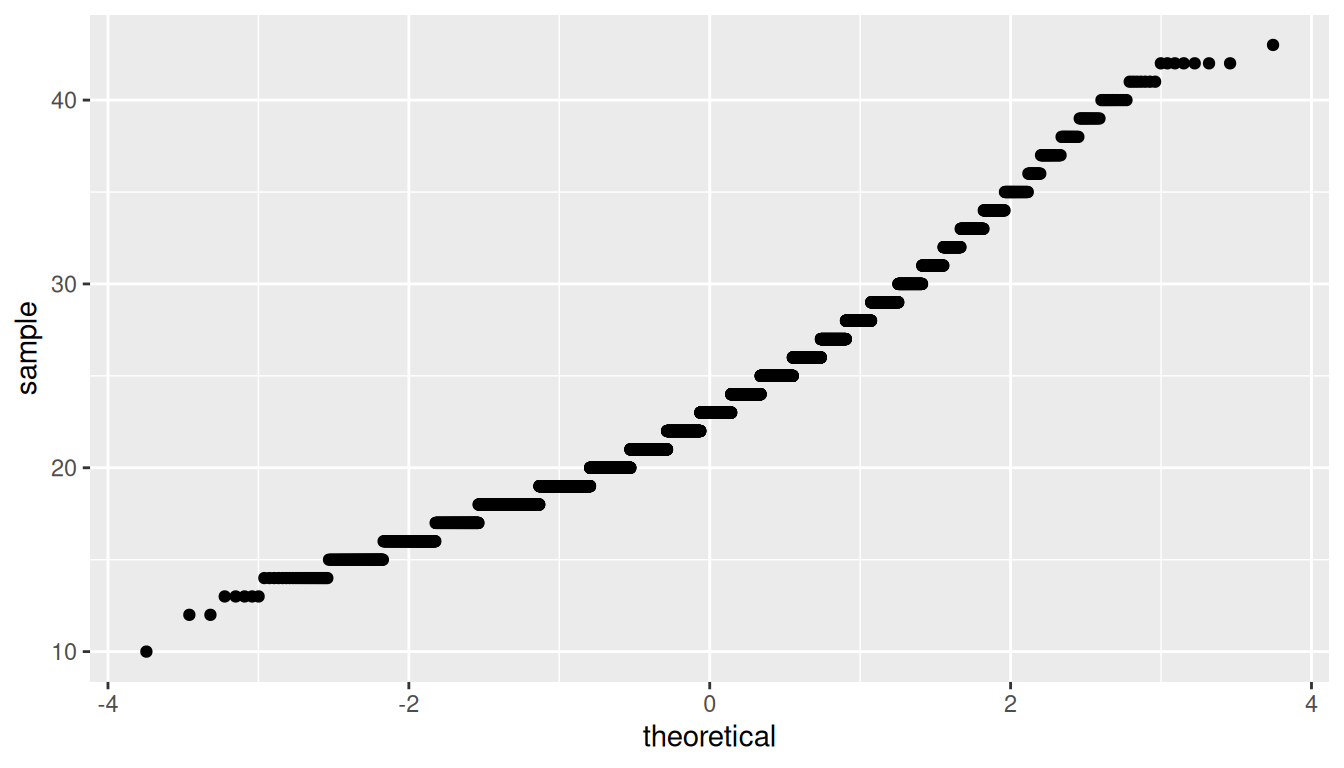## B.2 One mean

### B.2.1 Problem statement

The National Survey of Family Growth conducted by the Centers for Disease Control gathers information on family life, marriage and divorce, pregnancy, infertility, use of contraception, and men’s and women’s health. One of the variables collected on this survey is the age at first marriage. 5,534 randomly sampled US women between 2006 and 2010 completed the survey. The women sampled here had been married at least once. Do we have evidence that the mean age of first marriage for all US women from 2006 to 2010 is greater than 23 years? (Tweaked a bit from Diez, Barr, and Çetinkaya-Rundel 2014 [Chapter 4])

### B.2.2 Competing hypotheses

#### In words

• Null hypothesis: The mean age of first marriage for all US women from 2006 to 2010 is equal to 23 years.
• Alternative hypothesis: The mean age of first marriage for all US women from 2006 to 2010 is greater than 23 years.

#### In symbols (with annotations)

• $$H_0: \mu = \mu_{0}$$, where $$\mu$$ represents the mean age of first marriage for all US women from 2006 to 2010 and $$\mu_0$$ is 23.
• $$H_A: \mu > 23$$

#### Set $$\alpha$$

It’s important to set the significance level before starting the testing using the data. Let’s set the significance level at 5% here.

### B.2.3 Exploring the sample data

age_at_marriage <- read_csv("https://moderndive.com/data/ageAtMar.csv")
age_summ <- age_at_marriage %>%
summarize(
sample_size = n(),
mean = mean(age),
sd = sd(age),
minimum = min(age),
lower_quartile = quantile(age, 0.25),
median = median(age),
upper_quartile = quantile(age, 0.75),
max = max(age)
)
kable(age_summ) %>%
kable_styling(
font_size = ifelse(is_latex_output(), 10, 16),
latex_options = c("hold_position")
)
sample_size mean sd minimum lower_quartile median upper_quartile max
5534 23.4 4.72 10 20 23 26 43

The histogram below also shows the distribution of age.

ggplot(data = age_at_marriage, mapping = aes(x = age)) +
geom_histogram(binwidth = 3, color = "white")The observed statistic of interest here is the sample mean:

x_bar <- age_at_marriage %>%
specify(response = age) %>%
calculate(stat = "mean")
x_bar
# A tibble: 1 x 1
stat
<dbl>
1 23.4402

We are looking to see if the observed sample mean of 23.44 is statistically greater than $$\mu_0 = 23$$. They seem to be quite close, but we have a large sample size here. Let’s guess that the large sample size will lead us to reject this practically small difference.

#### Bootstrapping for hypothesis test

In order to look to see if the observed sample mean of 23.44 is statistically greater than $$\mu_0 = 23$$, we need to account for the sample size. We also need to determine a process that replicates how the original sample of size 5534 was selected.

We can use the idea of bootstrapping to simulate the population from which the sample came and then generate samples from that simulated population to account for sampling variability. Recall how bootstrapping would apply in this context:

1. Sample with replacement from our original sample of 5534 women and repeat this process 10,000 times,
2. calculate the mean for each of the 10,000 bootstrap samples created in Step 1.,
3. combine all of these bootstrap statistics calculated in Step 2 into a boot_distn object, and
4. shift the center of this distribution over to the null value of 23. (This is needed since it will be centered at 23.44 via the process of bootstrapping.)
set.seed(2018)
null_distn_one_mean <- age_at_marriage %>%
specify(response = age) %>%
hypothesize(null = "point", mu = 23) %>%
generate(reps = 10000) %>%
calculate(stat = "mean")
null_distn_one_mean %>% visualize()We can next use this distribution to observe our $$p$$-value. Recall this is a right-tailed test so we will be looking for values that are greater than or equal to 23.44 for our $$p$$-value.

null_distn_one_mean %>%
visualize(obs_stat = x_bar, direction = "greater")##### Calculate $$p$$-value
pvalue <- null_distn_one_mean %>%
get_pvalue(obs_stat = x_bar, direction = "greater")
pvalue
# A tibble: 1 x 1
p_value
<dbl>
1       0

So our $$p$$-value is 0 and we reject the null hypothesis at the 5% level. You can also see this from the histogram above that we are far into the tail of the null distribution.

#### Bootstrapping for confidence interval

We can also create a confidence interval for the unknown population parameter $$\mu$$ using our sample data using bootstrapping. Note that we don’t need to shift this distribution since we want the center of our confidence interval to be our point estimate $$\bar{x}_{obs} = 23.44$$.

boot_distn_one_mean <- age_at_marriage %>%
specify(response = age) %>%
generate(reps = 10000) %>%
calculate(stat = "mean")
ci <- boot_distn_one_mean %>%
get_ci()
ci
# A tibble: 1 x 2
2.5% 97.5%
<dbl>   <dbl>
1 23.3148 23.5669
boot_distn_one_mean %>%
visualize(endpoints = ci, direction = "between")We see that 23 is not contained in this confidence interval as a plausible value of $$\mu$$ (the unknown population mean) and the entire interval is larger than 23. This matches with our hypothesis test results of rejecting the null hypothesis in favor of the alternative ($$\mu > 23$$).

Interpretation: We are 95% confident the true mean age of first marriage for all US women from 2006 to 2010 is between 23.315 and 23.567.

#### Check conditions

Remember that in order to use the shortcut (formula-based, theoretical) approach, we need to check that some conditions are met.

1. Independent observations: The observations are collected independently.

The cases are selected independently through random sampling so this condition is met.

2. Approximately normal: The distribution of the response variable should be normal or the sample size should be at least 30.

The histogram for the sample above does show some skew.

The Q-Q plot below also shows some skew.

ggplot(data = age_at_marriage, mapping = aes(sample = age)) +
stat_qq()The sample size here is quite large though ($$n = 5534$$) so both conditions are met.

#### Test statistic

The test statistic is a random variable based on the sample data. Here, we want to look at a way to estimate the population mean $$\mu$$. A good guess is the sample mean $$\bar{X}$$. Recall that this sample mean is actually a random variable that will vary as different samples are (theoretically, would be) collected. We are looking to see how likely is it for us to have observed a sample mean of $$\bar{x}_{obs} = 23.44$$ or larger assuming that the population mean is 23 (assuming the null hypothesis is true). If the conditions are met and assuming $$H_0$$ is true, we can “standardize” this original test statistic of $$\bar{X}$$ into a $$T$$ statistic that follows a $$t$$ distribution with degrees of freedom equal to $$df = n - 1$$:

$T =\dfrac{ \bar{X} - \mu_0}{ S / \sqrt{n} } \sim t (df = n - 1)$

where $$S$$ represents the standard deviation of the sample and $$n$$ is the sample size.

##### Observed test statistic

While one could compute this observed test statistic by “hand”, the focus here is on the set-up of the problem and in understanding which formula for the test statistic applies. We can use the t_test() function to perform this analysis for us.

t_test_results <- age_at_marriage %>%
t_test(
formula = age ~ NULL,
alternative = "greater",
mu = 23
)
t_test_results
# A tibble: 1 x 6
statistic  t_df     p_value alternative lower_ci upper_ci
<dbl> <dbl>       <dbl> <chr>          <dbl>    <dbl>
1   6.93570  5533 2.25216e-12 greater      23.3358      Inf

We see here that the $$t_{obs}$$ value is 6.936.

#### Compute $$p$$-value

The $$p$$-value—the probability of observing an $$t_{obs}$$ value of 6.936 or more in our null distribution of a $$t$$ with 5533 degrees of freedom—is essentially 0.

#### State conclusion

We, therefore, have sufficient evidence to reject the null hypothesis. Our initial guess that our observed sample mean was statistically greater than the hypothesized mean has supporting evidence here. Based on this sample, we have evidence that the mean age of first marriage for all US women from 2006 to 2010 is greater than 23 years.

#### Confidence interval

t.test(
x = age_at_marriage$age, alternative = "two.sided", mu = 23 )$conf
 23.3 23.6
attr(,"conf.level")
 0.95

### B.2.6 Comparing results

Observing the bootstrap distribution that were created, it makes quite a bit of sense that the results are so similar for traditional and non-traditional methods in terms of the $$p$$-value and the confidence interval since these distributions look very similar to normal distributions. The conditions also being met (the large sample size was the driver here) leads us to better guess that using any of the methods whether they are traditional (formula-based) or non-traditional (computational-based) will lead to similar results.

### References

Diez, David M, Christopher D Barr, and Mine Çetinkaya-Rundel. 2014. Introductory Statistics with Randomization and Simulation. First. Scotts Valley, CA: CreateSpace Independent Publishing Platform. https://www.openintro.org/stat/textbook.php?stat_book=isrs.